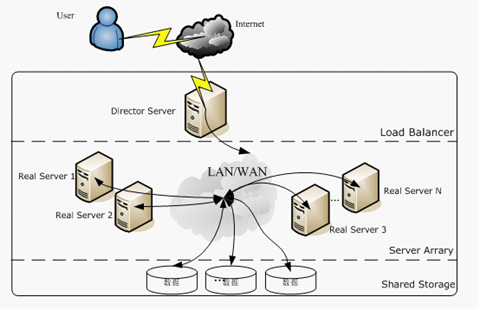# 机器学习算法之朴素贝叶斯

## 贝叶斯定理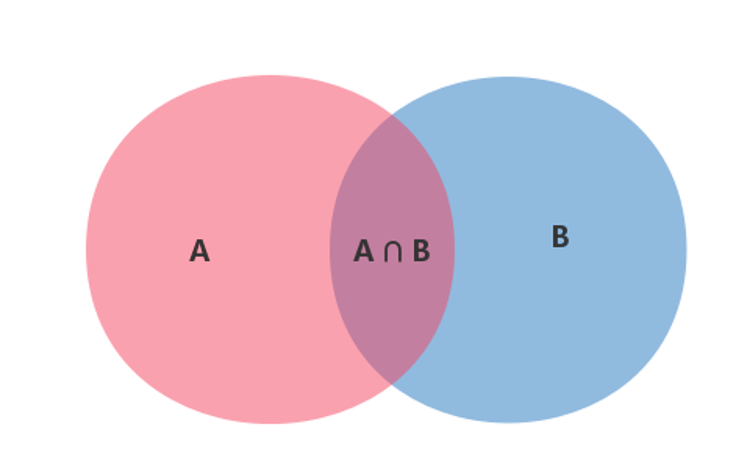$$P(A | B)=\frac{P(A \cap B)}{P(B)}$$

$$P(A | B)=\frac{P(B | A) P(A)}{P(B)}$$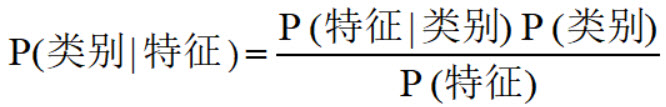## 朴素贝叶斯分类器

$$\mathop{argmax}_{y} P(y|x)$$

$$P(y|x_1,…,x_n) = \frac{P(y)P(x_1,…,x_n|y)}{P(x_1,…,x_n)}$$

• $P(y)$是先验概率，可以直接根据数据集计算出来。
• $P(x_1,…,x_n)$如何计算？
• $P(x_1,…,x_n|y)$如何计算?

$$P(XY|Z) = P(X|YZ)P(Y|Z)$$

$$P(UVWX|YZ) = P(U|VWXYZ)P(V|WXYZ)P(W|XYZ)P(X|YZ)P(Y|Z)$$

$$P(x_1,…,x_n|y) = P(x_1|x_2,…,x_n,y)P(x_2|x_3,…,x_n,y)…P(x_n|y)$$

$$P(x_i|y,x_1,…,x_{i-1},x_{i+1},…,x_n) = P(x_i|y)$$

$$P(x_1,…,x_n|y) = P(x_1|x_2,…,x_n,y)P(x_2|x_3,…,x_n,y)…P(x_n|y) =P(x_1|y)P(x_2|y)…P(x_n|y) = \frac{P(y)\prod_{i=1}^n P(x_i|y)}{P(x_1,…,x_n)}$$

$$P(x_1,…,x_n|y) \propto P(y)\prod_{i=1}^n P(x_i|y)$$

$$\hat y= \mathop{argmax}_{y} P(y|x)\prod_{i=1}^n P(x_i|y)$$

### 高斯朴素贝叶斯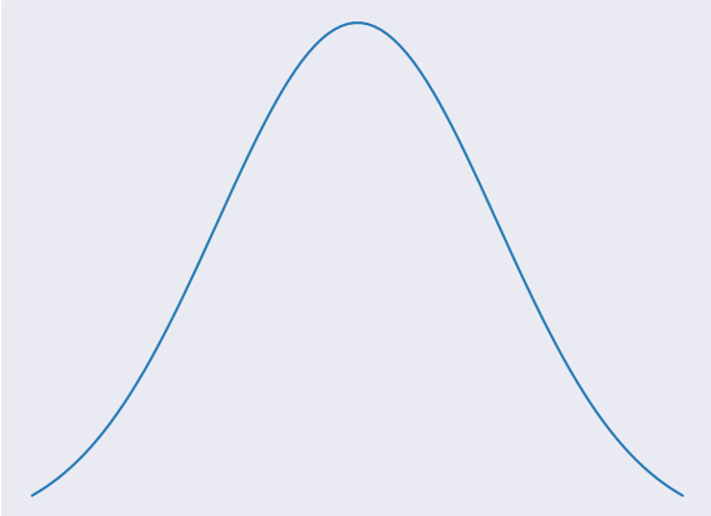$$P(y_k|X) = = \frac{P(y_k)\prod_{i=1}^{N}P(a_i|y_k)}{P(X)}$$

$$P（a_i|y_k） = \frac{1}{2\pi(\sigma_{yk})^2}*e^-\frac{(a_i-\mu_{yk})^2}{2(\sigma_{yk})^2}$$

SKlearn中的方法：

class sklearn.naive_bayes.GaussianNB(priors=None)
• priors:先验概率大小，如果没有给定，模型则根据样本数据自己计算（利用极大似然法）。

from sklearn.datasets import load_iris
from sklearn.model_selection import train_test_split
from sklearn.naive_bayes import GaussianNB

X_train, X_test, y_train, y_test = train_test_split(X, y, test_size=0.5, random_state=0)
gnb = GaussianNB()
y_pred = gnb.fit(X_train, y_train).predict(X_test)
print("Number of mislabeled points out of a total %d points : %d" % (X_test.shape, (y_test != y_pred).sum()))


### 多项式分布贝叶斯

$$P（a_i|y_k） = \frac{N(y_k,a_i)}{N(y_k)}$$

SKlearn中的方法：

class sklearn.naive_bayes.MultinomialNB(alpha=1.0, fit_prior=True, class_prior=None)
• alpha:先验平滑因子，默认等于1，当等于1时表示拉普拉斯平滑。
• fit_prior:是否去学习类的先验概率，默认是True
• class_prior:各个类别的先验概率，如果没有指定，则模型会根据数据自动学习， 每个类别的先验概率相同，等于类标记总个数N分之一。

from sklearn.datasets import load_iris
from sklearn.model_selection import train_test_split
from sklearn.naive_bayes import ComplementNB

X_train, X_test, y_train, y_test = train_test_split(X, y, test_size=0.5, random_state=0)
cnb = ComplementNB()
y_pred = cnb.fit(X_train, y_train).predict(X_test)
print("Number of mislabeled points out of a total %d points : %d" % (X_test.shape, (y_test != y_pred).sum()))


### 伯努利朴素贝叶斯

• 当特征值$x_i=1$时，$P(x_i|y)=P(x_i=1|y)$
• 当特征值$x_i=0$时，$P(x_i|y)=1-P(x_i=1|y)$

SKlearn中的方法：

class sklearn.naive_bayes.BernoulliNB(alpha=1.0, binarize=0.0, fit_prior=True, class_prior=None)
• alpha:平滑因子，与多项式中的alpha一致。
• binarize:样本特征二值化的阈值，默认是0。如果不输入，则模型会认为所有特征都已经是二值化形式了；如果输入具体的值，则模型会把大于该值的部分归为一类，小于的归为另一类。
• fit_prior:是否去学习类的先验概率，默认是True
• class_prior:各个类别的先验概率，如果没有指定，则模型会根据数据自动学习， 每个类别的先验概率相同，等于类标记总个数N分之一。

from sklearn.datasets import load_iris
from sklearn.model_selection import train_test_split
from sklearn.naive_bayes import BernoulliNB

X_train, X_test, y_train, y_test = train_test_split(X, y, test_size=0.5, random_state=0)
bnb = BernoulliNB()
y_pred = bnb.fit(X_train, y_train).predict(X_test)
print("Number of mislabeled points out of a total %d points : %d" % (X_test.shape, (y_test != y_pred).sum()))


## 朴素贝叶斯算法优缺点

• 朴素贝叶斯模型发源于古典数学理论，有稳定的分类效率
• 对小规模的数据表现很好，能个处理多分类任务，适合增量式训练，尤其是数据量超出内存时，我们可以一批批的去增量训练
• 对缺失数据不太敏感，算法也比较简单，常用于文本分类
• 算法逻辑简单,易于实现，分类过程中时空开销小

• 理论上，朴素贝叶斯模型与其他分类方法相比具有最小的误差率。但是实际上并非总是如此，这是因为朴素贝叶斯模型给定输出类别的情况下，假设属性之间相互独立，这个假设在实际应用中往往是不成立的，在属性个数比较多或者属性之间相关性较大时，分类效果不好。而在属性相关性较小时，朴素贝叶斯性能最为良好。对于这一点，有半朴素贝叶斯之类的算法通过考虑部分关联性适度改进
• 需要知道先验概率，且先验概率很多时候取决于假设，假设的模型可以有很多种，因此在某些时候会由于假设的先验模型的原因导致预测效果不佳
• 由于我们是通过先验和数据来决定后验的概率从而决定分类，所以分类决策存在一定的错误率
• 对输入数据的表达形式很敏感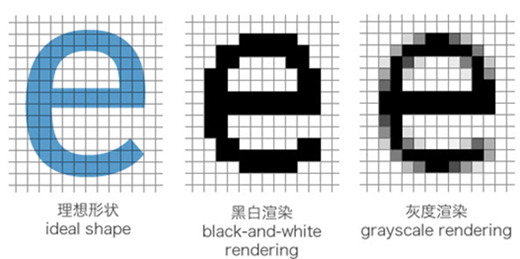##### 网站与APP开发中的字体设置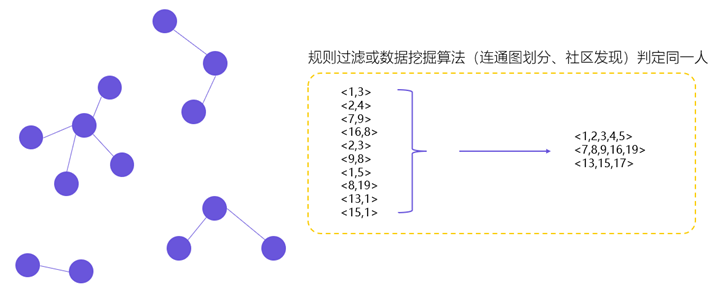##### 用户体系搭建之ID-Mapping